# Diffusion¶

$$\frac{\partial u}{\partial t} = \frac{\partial}{\partial x}D\frac{\partial u}{\partial x}$$
In :
%matplotlib inline
from pylab import *
from numpy import *

In :
#dimensions of the computational domain:
maxx = 10.
maxt = 1.

#difffusivity
D = 0.5


We discretize the simulation domain: $$t_n = t_0 + n\Delta t$$ $$x_i = x_0 + i\Delta x$$ and seek for the numerical approximation $U^n_i$ to the actual solution $U(t_n, x_i)$ on the grid points

In :
#discretization parameters
nx = 100   # number of unknown grid points in spatial direction
SC = 1.0  # stability criterion SC = 2*D*dt/dx^2, for FTCS should be SC <= 1

def diffusion_init(maxx, maxt, D, nx, SC):
#choose time step according to CFL condition
dx = maxx/(nx+1)
dt = SC*dx**2/(2*D)
nt = int(maxt/dt)+1

#define array for storing the solution
U = zeros((nt, nx+2))

x = arange(nx+2)*dx
t = arange(nt)*dt
return U, dx, dt, x, t


To obtain an explicit scheme for propagating the solution in time, we may replace the derivatives with forward differences in time and central differences in space (FTCS), assuming D=const. $$\frac{U^{n+1}_i-U^n_i}{\Delta t} = D\frac{U^n_{i+1}-2U^n_i+U^n_{i-1}}{\Delta x^2}$$ This leads to a simple explicit formula for $U^{n+1}_i$ $$U^{n+1}_i = U^n_i + \frac{D\Delta t}{\Delta x^2}(U^n_{i+1}-2U^n_i+U^n_{i-1})$$

In :
def diffusion_solve(U, dx, dt, nx, nt, D):
xint = arange(1, nx+1)
for it in range(0,nt-1):
U[it+1,xint] = U[it,xint] + D*dt/dx**2*(U[it,xint+1] - 2*U[it, xint] + U[it, xint-1])


Let's try to propagate a square initial condition

In :
U, dx, dt, x, t = diffusion_init(maxx, maxt, D, nx, SC=0.9)
U[0,:] = 0.
U[0, (x<maxx*0.4) & (x>maxx*0.2)] = 1.
plot(U[0,:])
ylim([0,1.1])

Out:
(0, 1.1)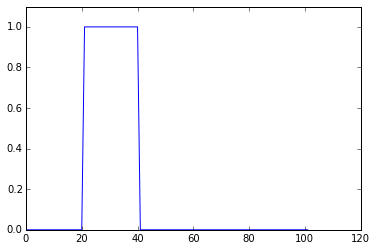In :
diffusion_solve(U, dx, dt, nx, len(t), D)
pcolormesh(U, rasterized=True, vmin=-1, vmax=1)

Out:
<matplotlib.collections.QuadMesh at 0x7f6952f01f60>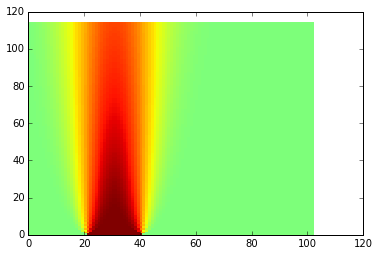The time step limit seems to be to strict, let's try increasing the time step a bit

In :
U, dx, dt, x, t = diffusion_init(maxx, maxt, D, nx, SC*1.03)
U[0,:] = 0.
U[0, (x<maxx*0.4) & (x>maxx*0.2)] = 1.

In :
diffusion_solve(U, dx, dt, nx, len(t), D)
pcolormesh(U, rasterized=True, vmin=-1, vmax=1)

Out:
<matplotlib.collections.QuadMesh at 0x7f6952ef7898>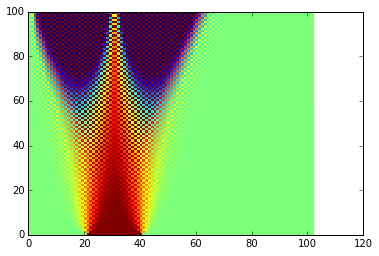Obviously, the stability condition is there for a reason :). As usual, implicit methods come to the rescue. Implicit differencing leads to $$\frac{U^{n+1}_i-U^n_i}{\Delta t} = D\frac{U^{n+1}_{i+1}-2U^{n+1}_i+U^{n+1}_{i-1}}{\Delta x^2}$$ Substituting $\alpha=\frac{D\Delta t}{\Delta x^2}$ and collecting the advanced values on left side yields $$-\alpha U^{n+1}_{i+1}+(2\alpha + 1)U^{n+1}_i-\alpha U^{n+1}_{i-1} = U^n_i$$ Or expressing in vectorized form, where $K$ is the matrix of second differences: $$U^{n+1}_i-U^n_i = \alpha K U^{n+1}$$ $$({1}-\alpha K) U^{n+1}=U^n$$

In :
from scipy.sparse import dia_matrix, eye
from scipy.sparse.linalg import factorized
def d2matrix(nelem):
elements = ones((3,nelem))
elements[1,:] *= -2
return dia_matrix((elements, [-1,0,1]), shape=(nelem,nelem)).tocsc()

def diffusion_solve_implicit(U, dx, dt, nx, nt, D):
alpha = D*dt/dx**2
d2x = eye(nx)-d2matrix(nx)*alpha
xint = arange(1, nx+1)
LU = factorized(d2x.tocsc())
for it in range(0,nt-1):
U[it+1,xint] = LU(U[it,xint])

In :
U, dx, dt, x, t = diffusion_init(maxx, maxt, D, nx, SC*10.)
U[0,:] = 0.
U[0, (x<maxx*0.4) & (x>maxx*0.2)] = 1.
#U[0,:] = sin(x*5)

In :
diffusion_solve_implicit(U, dx, dt, nx, len(t), D)
pcolormesh(U, rasterized=True, vmin=-1, vmax=1)

Out:
<matplotlib.collections.QuadMesh at 0x7f6952df6390>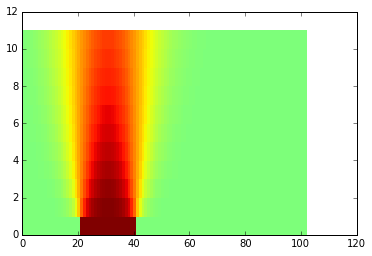Much longer time steps can now be used without compromising stability. In analogy to ODE methods, the previous methods correspond to explicit and implicit Euler methods. We may develop a method which is second order in time and space by proper time-centering: $$\frac{U^{n+1}_i-U^n_i}{\Delta t} = \frac{D}{2}\left( \frac{U^{n+1}_{i+1}-2U^{n+1}_i+U^{n+1}_{i-1}}{\Delta x^2}+ \frac{U^{n}_{i+1}-2U^{n}_i+U^{n}_{i-1}}{\Delta x^2}\right)$$ Again, substituting $\alpha=\frac{D\Delta t}{\Delta x^2}$ and collecting the advanced values on left side yields $$-\alpha U^{n+1}_{i+1}+(2\alpha + 2)U^{n+1}_i-\alpha U^{n+1}_{i-1} = -\alpha U^{n}_{i+1}+(2\alpha + 2)U^{n}_i-\alpha U^{n}_{i-1}$$ Or expressing in vectorized form, where $K$ is the matrix of second differences: $$U^{n+1}_i-U^n_i = \frac{\alpha}{2} K (U^{n+1}+U^n)$$ $$({1}-\frac{\alpha}{2} K) U^{n+1}=(1+\frac{\alpha}{2}K) U^n$$

In :
def diffusion_solve_CN(U, dx, dt, nx, nt, D):
alpha2 = D*dt/dx**2*0.5
M1 = eye(nx)-d2matrix(nx)*alpha2
M2 = eye(nx)+d2matrix(nx)*alpha2
LU = factorized(M1.tocsc())
for it in range(0,nt-1):
U[it+1,1:-1] = LU(M2.dot(U[it,1:-1]))

In :
diffusion_solve_CN(U, dx, dt, nx, len(t), D)
pcolormesh(U, rasterized=True, vmin=-1, vmax=1.0)

Out:
<matplotlib.collections.QuadMesh at 0x7f6952d547b8>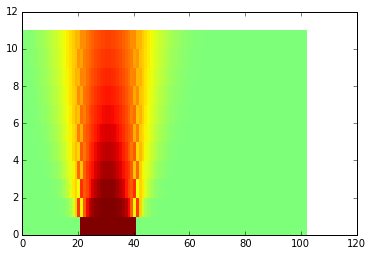What about other boundary conditions than 0 Dirichlet? In explicit schemes, the implementation is trivial. Otherwise, we need to put the BC to the RHS. For example nonzero Dirichlet BC conditions result:

In :


In :
def diffusion_solve_implicit(U, dx, dt, nx, nt, D):
alpha = D*dt/dx**2
d2x = eye(nx)-d2matrix(nx)*alpha
LU = factorized(d2x.tocsc())
for it in range(0,nt-1):
b = U[it,1:-1]
b[[0,-1]] += alpha*U[it+1,[0,-1]]
#U[it+1,1:-1] = LU(U[it,1:-1])
U[it+1,1:-1] = LU(b)

def diffusion_solve_CN(U, dx, dt, nx, nt, D):
alpha2 = D*dt/dx**2*0.5
M1 = eye(nx)-d2matrix(nx)*alpha2
M2 = eye(nx)+d2matrix(nx)*alpha2
LU = factorized(M1.tocsc())
print(U[0,0]+U[0+1,0])
for it in range(0,nt-1):
b = M2.dot(U[it,1:-1])
b[[0,-1]] += alpha2*(U[it,[0,-1]]+U[it+1,[0,-1]])
U[it+1,1:-1] = LU(b)


Model of heat conduction across a rod. Note the "huge" time step

In :
U, dx, dt, x, t = diffusion_init(maxx, maxt*100, D, nx, SC*40)
U[:,0] = 1.

In :
diffusion_solve_CN(U, dx, dt, nx, len(t), D)
pcolormesh(U, rasterized=True, vmin=-0, vmax=1)

2.0

Out:
<matplotlib.collections.QuadMesh at 0x7f6952d3be80>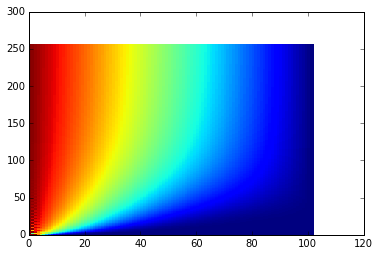In :
diffusion_solve_implicit(U, dx, dt, nx, len(t), D)
pcolormesh(U, rasterized=True, vmin=-0, vmax=1)

Out:
<matplotlib.collections.QuadMesh at 0x7f6952d173c8>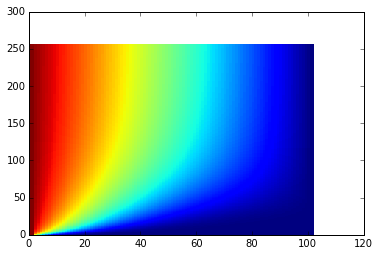Neumann BC

In :
def diffusion_solve_implicit_neumann(U, dx, dt, nx, nt, D, NBC = [0., 0.]):
alpha = D*dt/dx**2
n_unknowns = nx + 2
d2x = eye(n_unknowns)-d2matrix(n_unknowns)*alpha
d2x[0, 1] -= alpha
d2x[-1, -2] -= alpha
LU = factorized(d2x.tocsc())
for it in range(0,nt-1):
b = U[it,:]
b[[0,-1]] -= alpha*2*dx*array(NBC)
U[it+1,:] = LU(b)

In [ ]:
U, dx, dt, x, t = diffusion_init(maxx, maxt*50, D, nx, SC*20)
U[0,:] = 0.
U[0, (x<maxx*0.4) & (x>maxx*0.2)] = 1.

In [ ]:
diffusion_solve_implicit_neumann(U, dx, dt, nx, len(t), D)
pcolormesh(U, rasterized=True, vmin=-0, vmax=.5)

In [ ]: